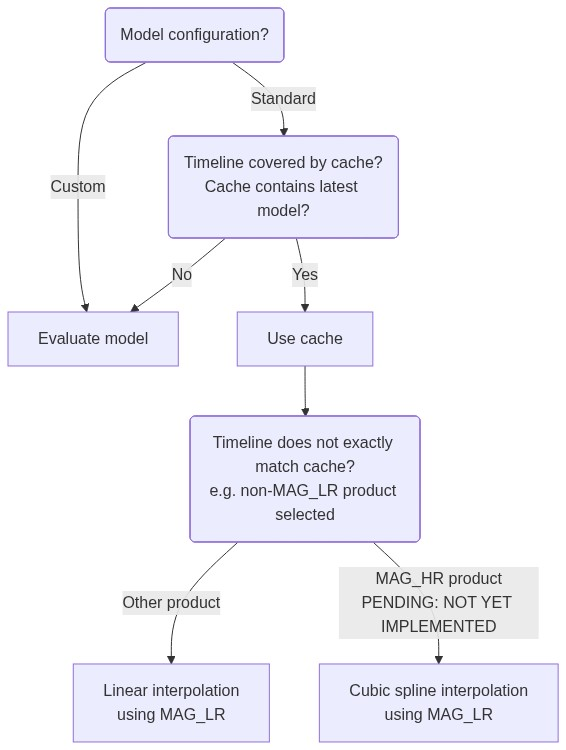# Geomagnetic model handling#

## Model evaluation#

The geomagnetic models provided by VirES are all based on spherical harmonics, though they differ in their parameterisation and time-dependence. They provide predictions of the different geomagnetic field sources (e.g. core, lithosphere, ionosphere, magnetosphere) and are generally valid near to Earth’s surface (i.e. at ground level and in LEO). To select appropriate models for your use case, you should refer to the scientific publications related to these models.

In VirES, we provide model evaluations calculated at the same sample points as the data products. This means that the spherical harmonic expansion is made at the time and location of each datapoint (e.g. in the case of `MAGx_LR`, at every second). The main purpose is to provide the data-model residuals, or magnetic perturbations, useful for studying the external magnetic field, typically ionospheric in origin.

Pending: In a future VirES release, some interpolation will be used to provide the magnetic model predictions along the 50Hz `MAGx_HR` products (to improve speed). The predictions at the 1Hz (`MAGx_LR`) locations will be used and a cubic interpolation performed to provide the predictions at the 50Hz locations.

The magnetic data products provide the magnetic field vector as the parameter `B_NEC` in the NEC (North, East, Centre) frame, as well as the magnetic field intensity/magnitude (scalar), `F`. When also requesting models from VirES (by supplying the `models` kwarg to `viresclient.SwarmRequest.set_products()`), the corresponding model predictions will be returned in parameters named `B_NEC_<model-name>` or `F_<model-name>`. Alternatively, the data-model residual alone, named `B_NEC_res_<model-name>` or `F_res_<model-name>` can be returned directly by also supplying the kwarg `residuals=True`. Models should be provided as a list, like `models=["CHAOS", "IGRF"]`.

## Available models#

See Available parameters for Swarm / models for the list of available models.

You can use `viresclient.SwarmRequest.available_models()` and `viresclient.SwarmRequest.get_model_info()` to query the details of models.

## Composed and custom models#

When providing `models` to `viresclient.SwarmRequest.set_products()`, they can be customised:

Rename:
`models=["Model='CHAOS-Core'"]`
This will provide the `CHAOS-Core` model renamed to `Model`, so that the returned parameters will include `B_NEC_Model` instead of `B_NEC_CHAOS-Core`.
Compose (combine):
`models=["Model='CHAOS-Core' + 'CHAOS-Static'"]`
This sums together the contribution from `CHAOS-Core` and `CHAOS-Static` into a custom model called `Model`.
Customise:
`models=["Model='CHAOS-Core'(max_degree=20) + 'CHAOS-Static'(min_degree=21,max_degree=80)"]`
This limits the spherical harmonic degree used in the model calculation.

Note that single and double quotes are interchangeable, and must be used sometimes in order to enclose a model name and thus distinguish usage of a hyphen (`-`) in the model name from an arithmetic minus.

You can query information about your selected models using `viresclient.SwarmRequest.get_model_info()`:

```from viresclient import SwarmRequest

request = SwarmRequest()

request.get_model_info(
models=["Model='CHAOS-Core'(max_degree=20) + 'CHAOS-Static'(min_degree=21,max_degree=80)"]
)
```
```{'Model': {'expression': "'CHAOS-Core'(max_degree=20,min_degree=1) + 'CHAOS-Static'(max_degree=80,min_degree=21)",
'validity': {'start': '1997-02-07T05:23:17.067838Z',
'end': '2024-03-01T02:57:24.851521Z'},
'sources': ['CHAOS-7_static.shc',
'SW_OPER_MCO_SHA_2X_19970101T000000_20230807T235959_0715',
'SW_OPER_MCO_SHA_2X_20230808T000000_20240229T235959_0715']}}
```

## Model caching#

To speed up usage of commonly used expensive models, the server stores and uses a cache of some of the model values (so that they do not always need to be evaluated from scratch). This should happen transparently so you generally do not need to worry about it, but it may be helpful to understand when the cache might not be used, causing data requests to take longer.

Note

The caching mechanism can be bypassed (forcing direct evaluation of models) by supplying `ignore_cached_models=True` in `viresclient.SwarmRequest.set_products()`

Cached models (these are chosen as they are both expensive and commonly used):

```CHAOS-Static
MIO_SHA_2C-Primary
MIO_SHA_2C-Secondary
MLI_SHA_2C
```

The predictions for these models are cached only at the positions and times defined by the following products (i.e. low resolution magnetic products):

```SW_OPER_MAGx_LR_1B (x=A,B,C)
GRACE_x_MAG (x=A,B)
GFx_OPER_FGM_ACAL_CORR (x=1,2)
GO_MAG_ACAL_CORR
GO_MAG_ACAL_CORR_ML
CS_OPER_MAG
```

The logic describing when the cache is used is as follows:Custom configured models, e.g. `CHAOS-Static(max_degree=80)`, are not cached and must be evaluated directly.

Composed models, i.e. `Model = Model1 + Model2`, will use the cache for sub-models where available. For example, choosing `CHAOS-Core + CHAOS-Static` will make use of the cache for `CHAOS-Static` (an expensive model), but will directly evaluate `CHAOS-Core` (a cheap model), and combine the result. The same is true for alias models such as `CHAOS` (which equates to `CHAOS-Core + CHAOS-Static + CHAOS-MMA`).

When the source products or model are updated, the cache needs to be re-generated accordingly. This means means there is some delay before the cache is available again (while the changes are still being processed). In cases where the cache has been obsoleted, the system falls back to evaluating the model directly. In short, the caching mechanism prefers model consistency over performance.

## Model values through HAPI#

What is HAPI? See https://notebooks.vires.services/notebooks/02h1_hapi

When accessing magnetic datasets, there are additional HAPI parameters available:

```B_NEC_Model
F_Model
B_NEC_res_Model
F_res_Model
```

These give, respectively, vector and scalar magnetic model values and data-model residuals using the full CHAOS model (core + lithosphere + magnetosphere). These are provided through the cache as described above.